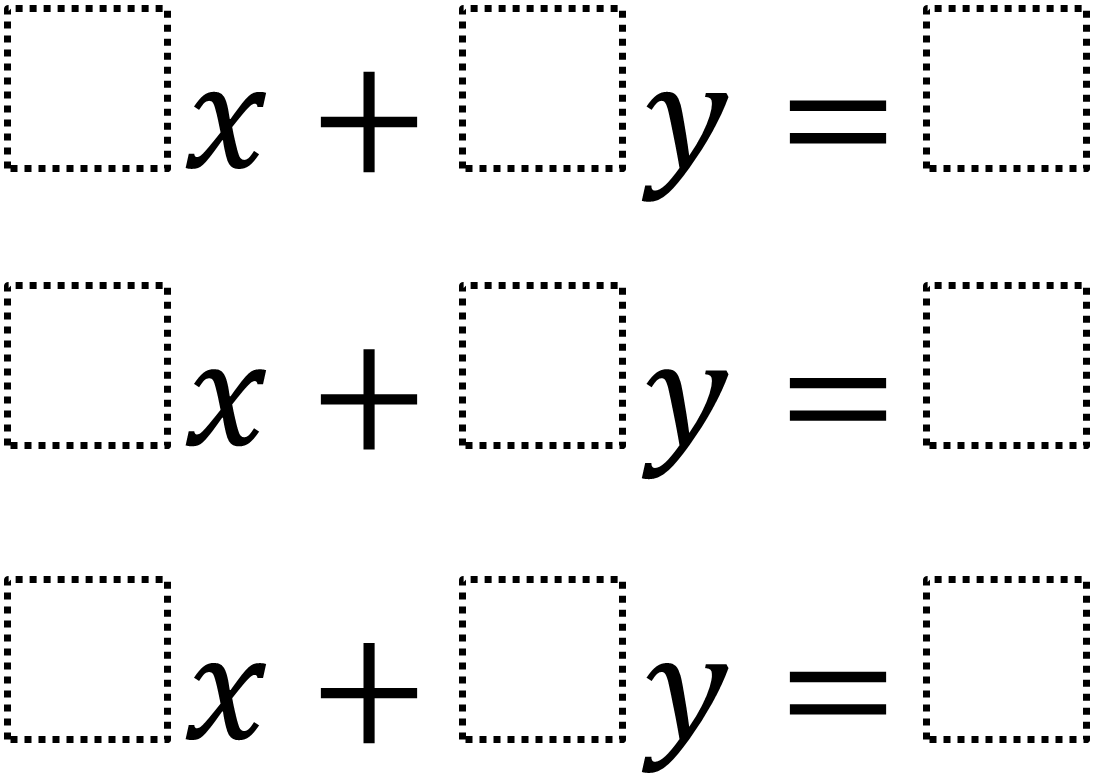# Parallel Lines and Transversals

Directions: Using the digits 1 to 9 at most one time each, fill in the boxes so that 2 of the lines are parallel and the third line is a transversal.### Hint

What do we know about the slopes of parallel lines and transversals?

### Answer

There are multiple answers but two of the lines must be parallel (have the same slope) and the third must have a different slope.  One solution is 6x + 3y = 9, 4x + 2y = 8, x + 7y = 5

Source: Shelli Foust

## Pythagorean Inequality

Directions: Using the digits 1 through 6 at most one time each, fill in the …